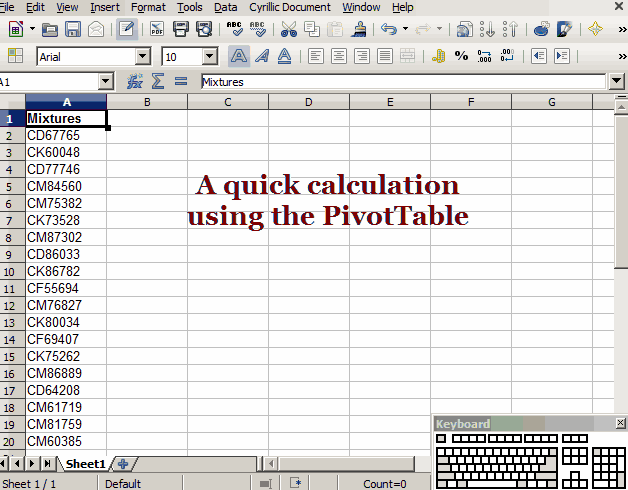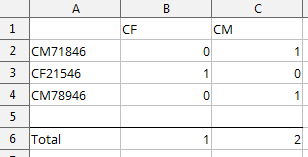# COUNTING TEXT IN COLUMNS

I have columns with a mixture of text and numbers eg

CM71846
CF21546
CM78946
CF12456
CF24566

How can I count the number of CF and number of CM please

@Nifty – If one of the answers below works well for you, please mark it as correct.

Thanks!

Thanks great help by all

If you have many text variations, you can use a pivot tableThere is a function to do it:

=COUNTIF(A1:A10;“CF.*”)

Please take a look in the help about the function and the regular expressions.

Sorry I tried that and for sum reason function would not work returns 0 all the time.

@Nifty regular expressions can be disabled in the (advanced) options/settings screen. Perhaps yours is disabled? CF.* would match anything containing CF, followed by any number of characters.I’m assuming that you only have one letter/number combination in each cell. Use the image above as a guide. In the example, paste this formula in cell B2:

``````=IF(LEFT(A2,2)="CF", 1, 0)
``````

and this one in C2:

``````=IF(LEFT(A2,2)="CM", 1, 0)
``````

Drag the cell handle of each of these cells down to fill the formulas down to the end of your data. Then sum columns B and C.